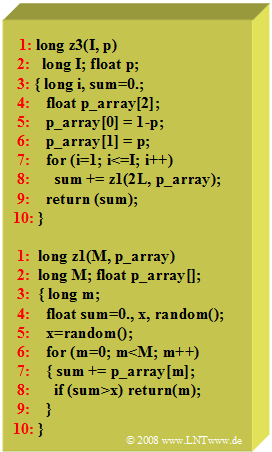# Exercise 2.7Z: C Program "z3"C program  $z3$  for generating
a binomial distribution

The C program  $z3$  given here successively generates a binomially distributed random variable with the characteristic variables  $I$  and  $p$.

• It uses the program  $z1$ already described and analyzed in  Exercise 2.7 .
• Assume that the program is called with parameters  $I = 4$  and  $p = 0.75$ .
• The first eight real-valued numbers  (all between zero and one)  generated by the random generator  $\text{random()}$  are:
$$\rm 0.75, \ 0.19, \ 0.43, \ 0.08, \ 0.99, \ 0.32, \ 0.53, \ 0.02.$$

Hints:

### Questions

1

Which of the following statements are true?

 $z3$  returns a binomially distributed random variable,  because several binary values are summed up. To pass parameters to the program  $z1$,  the array   $\text{p_array} = \big [1-p, \ \ p \big]$   is used. Passing of  $M=2$  must be done with  "$\rm 2L$",  because  $z1$  expects a long (data type) value.

2

What value will be output on the  first call  of  $z3$ ?

 $z3 \ = \$

3

What value will be output on the  second call  of  $z3$ ?

 $z3 \ = \$

### Solution

#### Solution

(1)  All three statements  are true.

(2)  The real-valued random numbers  $0.75$,  $0.19$,  $0.43$  and  $0.08$  are each compared with  $0.25$.

• This comparison results in the binary values  $1, \ 0, \ 1, \ 0$.
• This results in the sum  $\underline{z3 = 2}$  for the first call.

(3)  Analogous to the result of the subtask  (2)  the binary values  $1, \ 1, \ 1, \ 0$  now occur because of the random values  $0.99$,  $0.32$,  $0.53$  and  $0.02$  .

• This leads to the output value  $\underline{z3 = 3}$  (again the sum of binary values).